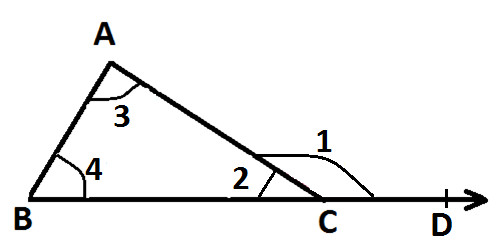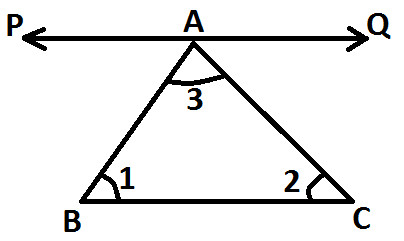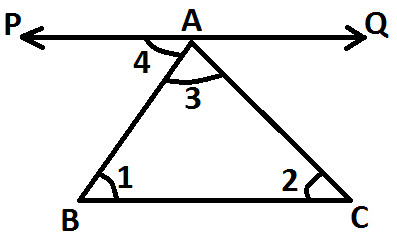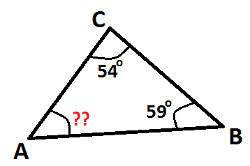Arithmetic
Arithmetic Progression
Associative Property
Averages
Brackets
Closure Property
Commutative Property
Conversion of Measurement Units
Cube Root
Decimal
Distributivity of Multiplication over Addition
Divisibility Principles
Equality
Exponents
Factors
Fractions
Fundamental Operations
H.C.F / G.C.D
Integers
L.C.M
Multiples
Multiplicative Identity
Multiplicative Inverse
Numbers
Percentages
Profit and Loss
Ratio and Proportion
Simple Interest
Square Root
Unitary Method
Algebra
Cartesian System
Order Relation
Polynomials
Probability
Standard Identities & their applications
Transpose
Geometry
Basic Geometrical Terms
Circle
Curves
Angles
Define Line, Line Segment and Rays
Non-Collinear Points
Parallelogram
Rectangle
Rhombus
Square
Three dimensional object
Trapezium
Triangle
Trigonometry
Trigonometry Ratios
Data-Handling
Arithmetic Mean
Frequency Distribution Table
Graphs
Median
Mode
Range

Videos
Solved Problems
Home >> Triangle >> Properties >> Angle Sum Property >>

## Angle Sum Property of Triangle

 Sum of Two Sides Angle Sum Property Angles opposite to equal sides of triangle are equal Angle opposite to longer side is greater Pythagoras Theorem Exterior Angle Property of a Triangle Mid point property of Triangle Triangles on same base & between same parallel lines

Angle Sum Property of a Triangle says that Sum of all the Angles of the Triangle is always equal to 180°.

For Better understanding of Angle Sum Property, study the following examples :-

Example 1 = Below diagram represent Triangle ABCIn the above diagram, Triangle ABC has
∠ A = 45°
∠ B = 90°
∠ C = 45°

Now as per the Angle Sum Property,
Sum of ∠ A, ∠ B & ∠ C has to be equal to 180°.

Lets Check the property :-
∠ A + ∠ B + ∠ C = 180°
Put the values of all angle and we get:
45° + 90° + 45° = 180°
180° = 180°

since L.H.S = R.H.S
Hence its proved that Sum of all the Angles of the Triangle is always equal to 180°.

Example 2 = Below diagram represent Triangle PQRIn the above diagram, Triangle ABC has
∠ P = 70°
∠ Q = 60°
∠ R = 50°

Now as per the Angle Sum Property,
Sum of ∠ P, ∠ Q & ∠ R has to be equal to 180°.

Lets Check the property :-
∠ P + ∠ Q + ∠ R = 180°
Put the values of all angle and we get:
70° + 60° + 50° = 180°
180° = 180°

since L.H.S = R.H.S
Hence its proved that Sum of all the Angles of the Triangle is always equal to 180°.

How to prove angle sum property of triangle:
There are several methods to prove angles sum property of triangle, but here we have explained three methods only.

Method 1: Prove angle sum property of triangle with the help of protractor
Draw a triangle and measure all its angles with the help of protractor.
Now add the measurement and you will notice that sum of these measurement is equal to 180° (ignore marginal error).
You can try this with few more triangles and you will notice that in all other triangles too; sum of measurement of all the angles is equal to 180°.

Hence, this prove angles sum property of triangle, which says that sum of all the angles of triangle is equals to 180°

Method 2: Prove angle sum property of triangle with the help of exterior angle property.

Before you study this method you are advised to read:
What is Exterior Angle Property of a Triangle ?
What is Linear Pair ?

Draw a triangle ABC and produce BC to D as shown in the following figure:Mark all the angles of triangles as ∠ 2, ∠ 3 & ∠ 4 as shown in the above figure.
Also, mark exterior angle as ∠ 1 as shown in the above figure.

Now as per Exterior Angle Property which says that exterior angle is equal to sum of interior opposite angles; we get:
∠ 1 = ∠ 4 + ∠ 3 .....(statement 1)

Also, ∠ 1 & ∠ 2 from linear pair, so we get:
∠ 1 + ∠ 2 = 180°

Put the Values of angles ∠ 1 from (statement 1) and we get:
∠ 4 + ∠ 3 + ∠ 2 = 180°
And ∠ 4, ∠ 3 & ∠ 2 all are the angles of triangle ABC.

Hence, Angle Sum Property is proved which says that Sum of all the angles of a triangle are equals to 180°

Method 3: Prove angle sum property of triangle with the principles of parallel lines cut by a transversal
Before you understand this method, you are advised to read:

What is Transversal Line ?
What are the Properties of Transversal ?
What is Linear Pair ?

In the below diagram we have a triangle ABC and we have to prove the angle sum property of triangle i.e. sum of it's angles is equal to 180°
Or we write it as:
∠ 1 + ∠ 2 + ∠ 3 = 180°Following are the steps to prove Angle Sum Property of Triangle:

• Draw a line PQ parallel to side BC which passes through the Vertex A (as shown below):• Line PQ is parallel to BC and AB is a transversal, we get:
∠ 1 and ∠ 4 are alternative interior (as shown below)And as per the property of transversal, If two parallel lines are cut by a transversal, then pair of alternative interior angles are equal. So we get:
∠ 1 = ∠ 4 ..... (Statement 1)

• Similarly, Line PQ is parallel to BC and AC is a transversal, we get:
∠ 2 and ∠ 5 are alternative interior angles (as shown below):And as per the property of transversal, "If two parallel lines are cut by a transversal, then pair of alternative interior angles are equal". So we get:
∠ 2 = ∠ 5 ..... (Statement 2)

Now, you can observe that Angle 3, Angle 4 and Angle 5 lies on the line PQ, thereby forming a Linear Pair, so we get:
∠ 4 + ∠ 3 +∠ 5 = 180°

As proved in statement 1 and 2 above (∠ 4 = ∠ 1 & ∠ 5 = ∠ 2), so we get:
∠ 1 + ∠ 3 + ∠ 2 = 180°

Or we can write it as:
∠ 1 + ∠ 2 + ∠ 3 = 180°

Hence, this proved angle sum property of triangle with the principles of parallel lines cut by a transversal

### Study More Solved Questions / Examples

 Find the measurement of ∠ A in the following figure:In a triangle PQR, ∠ P is equals to 50° and ∠ Q & ∠ R are equal. Find measurement of ∠ Q & ∠ R Angles of a triangle are in the ratio of 1:2:1. Find the measurement of all the angles.
• Copyright@2022 Algebraden.com (Math, Algebra & Geometry tutorials for school and home education)